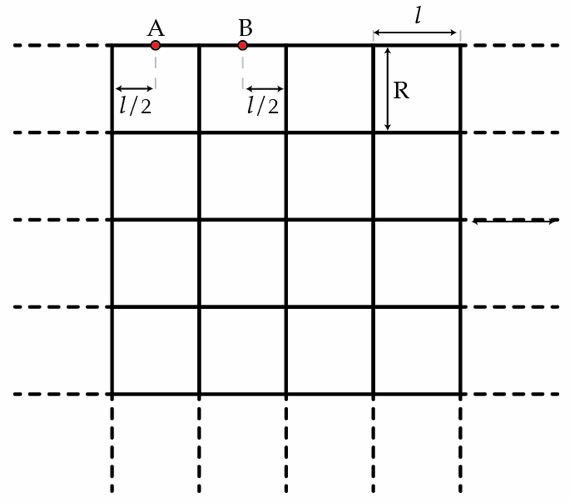# Semi-infinite grid

Suppose we build a semi-infinite grid with square cells as shown in the figure below. Each wire has a length $l$ and a resistance of $r=1~\Omega$. What is the equivalent resistance between the points A and B?×

Problem Loading...

Note Loading...

Set Loading...